## State-Space Modelling in Continues Time: Trend, Momentum, Anomalies, Regimes and Forecasting¶

This series of notebooks will cover the practical application of so called state-space models towards understanding market behaviour as well as the underlying market microstructure and how this new information can help us design profitable algorithmic trading models. I will mention right away that a purely theoretical description, while necessary to understand the topic in full, will most likely be counterproductive as it is can be very math heavy; instead I will try to build an intuitive understanding of the subject via a practical treatment of various ideas and concepts encountered within. That being said, mathematics are sometimes unavoidable and I will need to define certain ideas mathematically first before further explanation can be given. Finally, at this stage, this notebook is largely experimental and many of the ideas presented here, while indeed very promising, will require more work and testing before we can make use of them in a live scenario.

Before we can approach the subject at large we should first cover some basic concepts and explain our motivation for state-space modelling.

## Price as signal¶

A raw price series is a continues time series devoid of all other information but the price and volume of an asset, future, derivative or any other financial instrument. For illustrative purposes here is an hourly OHLC (open-high-low-close) chart of the BCH/BTC pair.

In :
import numpy as np
import pandas as pd
import matplotlib.pyplot as plt
from mpl_finance import candlestick2_ochl

_, ax = plt.subplots(figsize=(15,  5))
candlestick2_ochl(ax, s['open'], s['close'], s['high'], s['low'], width=2)
plt.show()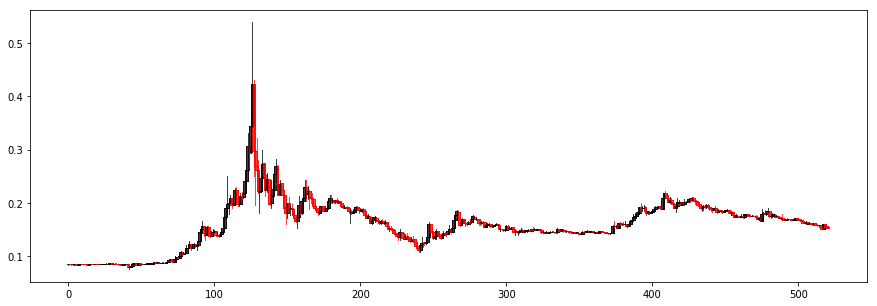We are interested in extracting additional information, importantly valuable information we can trade on, from this raw object.

Now, at first glance this seems like we are approaching the domain of Technical Analysis (TA). Note the capitalization, I'm not talking about technically assessing prices and price levels and so on, TA here means relying on so called "technical indicators" like the "RSI", "William's R%" and many, many other indicators which are purely influenced by historic price and volume levels to understand and model market behaviour. Many, including myself, view this type of approach as pseudo-scientific at best largely because TA is mostly interpretive and expressions like "You just didn't use it properly" or "The market is just TA resistant right now" and "He's really good at TA" are rather common.

While TA can't be dismissed outright (we would need to prove beyond any doubt that all of the 10k or so indicators are no better than random first), the TA approach itself or rather how it is practiced currently, should be safely dismissed as it is not objective and relies on subjective interpretation of indicators. In other words, it is more (much more) art than science. Practitioner A and Practitioner B trading the same currency pairing and relying on the same indicators are likely to interpret the generated buy/sell signals in vastly different ways. Designing an algorithm which will rely on technical indicators will thus require a subjective heuristic of sorts, making personal bias unavoidable...

Beyond that, one wouldn't be wrong in assuming that TA is inefficient purely because it is in such wide practice. Most TA forums boast hundreds of thousands of subscribers, yet, and of course this is purely anecdotal but seems rational enough an assumption, the vast majority are unable to outperform the market. The usual retort to that is "Well, you just don't know how to use it well" which we of course covered above.

#### Efficient Market Hypothesis¶

The Efficient Market Hypothesis (EMH) states that asset prices fully reflect all available information making it impossible to beat the market as no practitioner is able to either purchase undervalued assets or sell assets for inflated prices. Quoting from wikipedia

There are three variants of the hypothesis: "weak", "semi-strong", and "strong" form. The weak form of the EMH claims that prices on traded assets (e.g., stocks, bonds, or property) already reflect all past publicly available information. The semi-strong form of the EMH claims both that prices reflect all publicly available information and that prices instantly change to reflect new public information. The strong form of the EMH additionally claims that prices instantly reflect even hidden "insider" information.

Whatever view of the EMH is taken, it strongly implies that historic price information can not serve as a signal worth trading on as all of the information contained within is total and available to everyone else the moment it becomes available to us, thus it is arbitraged away by default.

#### A Random Walk¶

The "Random Walk Hypothesis" views the evolution of a price series subject to a random walk distribution. A random walk is a stochastic mathematical process that describes a path consisting of a series of random steps within a given mathematical subspace. This implies the inability to explain current or future price behaviour based on historic price behaviour alone. Below is a random walk model of a fictional asset's closing prices. The stochastic process behind our asset's value is a simple calculation based on consecutive digits of $\Pi$: increase when even, decrease if odd.

In :
from mpmath import mp

bp = 100.0
inc = 2.
mp.dps = 1000
closes = [bp]
for p in [inc if int(i) % 2 == 0 else -inc for i in str(mp.pi) if i.isdigit()]:
bp += p
closes.append(bp)
_, ax = plt.subplots(figsize=(15,  5))
plt.plot(closes)
plt.show()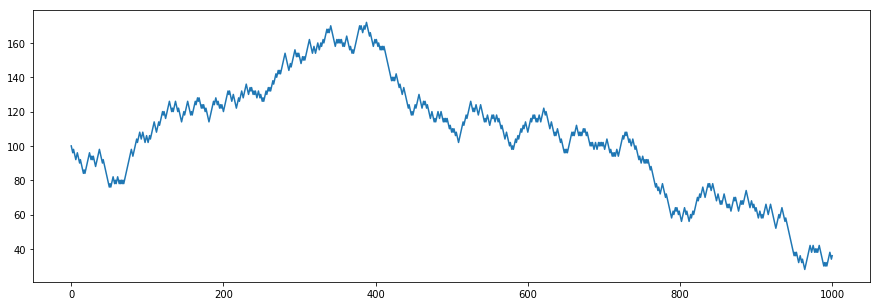Notice how the resulting series resembles the price behaviour of a real asset.

## The (largely) inefficient market¶

Both the EMH as well as the RWH assumptions of market behaviour are closely related and certainly worth a consideration at least.
However, is price behaviour truly random? More importantly, does EMH hold true no matter what?

#### A brief history of financial modelling¶

Modelling under the random walk distribution took root in the late 60s. Lorie and Hamilton in their 1973 paper on distributions of stock returns first came to the conclusion that "price has no memory", if an investors technique is conditioned on some ad hoc price configuration, there will be little value added because a random walk stock will give us no profitable clues about future prices. It was Fama who artfully formed and expanded the notion of random walk into what he popularized as the efficient market hypothesis in the mid 1970s. Such was the brilliance of Fama's paper that within no time at all the idea of the efficient and perfect market was firmly implanted in the brains of most financial economists, academics and econometricians, so much so that any divergent view would be akin to career suicide. Empirical findings disproving the EMH assumption or at least poking several large holes in the theory existed however never saw the light of day in a rather hostile environment.

Yet, where there is a will there is usually a way and by the early 80s there came a large volume of formal literature that discovered inefficiencies that could lead to abnormal returns if rigorously applied. The list includes size effect, January effect, value irregularities, momentum effect, etc.

Like in most sciences the road towards getting published was of course to operate under the formal assumption of EMH and thus all irregular inefficiencies were called "anomalies" it was also acknowledged that the discoveries were (1) historic and likely not repeatable (now that we know them) (2) inconclusive because of potential "risk misspecification" (3) lacking proper allocation of costs when formulating strategies.

It really took a new and emerging field, the field of behavioural finance to successfully argue against EMH and establish the ineffective market as a viable model. Psychologists and behavioural scientists formulated such notions as "herd behaviour in financial practitioners", "irrational exuberance", "under- and overreaction", "biased self-attribution" and many, many others. These and similar models effectively explained real market behaviour like economic bubbles, heteroskedastic shock, price drift and so on.

By the late 90s the market was assumed inefficient and ineffective by default.

A few notable models came out towards the end of the millennium. Daniel, Hirschleifer, and Subrahmanyam (DHS) (1998) assume that investors are overconfident about their private information, and their overconfidence increases gradually with the arrival of public information with biased self-attribution.

Hong and Stein (HS) (1999) make two assumptions: (1) investors are bounded rational, meaning that they have limited intellectual capacity and that they are rational in processing only a small subset of the available information; (2) information diffuses slowly across the population.

Barberis, Shleifer, and Vishny (BSV) (1998) suggest that investors exhibit two biases in updating their prior beliefs with public information: Conservatism and Representativeness. Conservatism states that investors are slow to change their beliefs in the face of new evidence; while representativeness is a biased understanding of probabilities, that is assessing the probability of an event by finding a "similar known" event and assuming that the probabilities will be similar.

The findings of Hong and Stein in particular will be of significant importance to us throughout the rest of this notebook.

#### A modern approach¶

Modern quantitative finance operates under the assumption of market inefficiency, which is attributed to investors irrationality as explained by behavioural finance and behavioural econometrics. That being said, it is very important to not fall into that basket of irrationality ourselves when designing and executing strategies. While it can be shown that past price behaviour does impact future price behaviour it is important to quantify this assumption and it is equally as important to design an objective interpretation to model this type of behaviour. In other words, the TA approach is still ill-conceived and ill-fitted to model a perfectly rational or a perfectly irrational market...

## Momentum and Trend¶

As mentioned above, HS's findings are of particular interest as they carry over certain implications about the relation between past and future price behaviour. One notable anomaly encountered in almost all financial time series is that of momentum. Quoting wikipedia

In finance, momentum is the empirically observed tendency for rising asset prices to rise further, and falling prices to keep falling. For instance, it was shown that stocks with strong past performance continue to outperform stocks with poor past performance in the next period with an average excess return of about 1% per month.

Momentum is an anomaly because there is no rational (rational as viewed through the EMH lens) explanation why an asset's past performance would impact its future value. Momentum can be short lived and long lived, it can trend upwards or downwards. Momentum, in most cases, suffers from reversal.

HS view momentum as influenced by mostly two factors. They specify two bounded rational agents - news-watchers and momentum traders themselves. Both are risk-averse, and their interactions set future prices. On the one hand, news-watchers exhibit similar behaviour to a typical fundamental manager in practice, observe some private information and ignore information in past and current prices. On the other hand, momentum traders condition their forecasts only on past price changes, and their forecast method is simple. The slow diffusion of information among news-watchers induces underreaction in the short run. Underreaction leads to positively autocorrelated returns - momentum continues. Upon observing this predictable return pattern, momentum traders condition their forecast only on past price changes and arbitrage the profit opportunity. Arbitrage activity eventually leads to overreaction in the longrun, creating dislocation between price and fundamentals. The reversal of price back to fundamental is the source of momentum reversal.

In simpler terms, momentum is in part motivated by our limited capacity to react and process new information, as new information arises it reaches more and more participants in a diffused manner rather than all at once. Participants are also slow to react and act upon this new information. This leads to a certain lag in price behaviour. While under the EMH assumption any information is instantly reflected in the price of an asset, in reality the slow diffusion of information leads to a rather prolonged period of adjustment and reflection.

Momentum is also motivated by momentum traders themselves. For example, a TA practitioner is betting on exploiting this phenomenon by gauging for momentum and longing when the price goes up while shorting when the price goes down. He might rely on something like the MACD (moving average convergence divergence) indicator to find a good spot for entry and exit. This becomes a self fulfilling prophecy of sorts as more and more momentum traders jump in to arbitrage this opportunity. Momentum continues to build stronger and finally collapses as the assets price grows (or falls) too divergent from its fundamental value leading to momentum reversal.

#### Strategy formulation¶

Taking the above into account we are able to formulate our first belief and strategy. Here presented in brief:

• If momentum exists, price at time $t_n$ can be viewed as strongly correlated to price at time $t_{n-1}$
• Price or price behaviour in the past can be viewed as a signal $S$
• The trend/momentum of price behaviour $Ph$ is influenced by $S + \epsilon_t$ the signal (past behaviour) and random, time-dependent noise
• Exploit momentum due to information diffusion by entering (or shorting) when it starts building
• Exploit momentum due to momentum reversal by entering (or shorting) when divergence from fundamental is strongest

Admittedly this is a rather uninvolved strategy, we simply bet on capturing momentum and reversal faster than the general market.

## Tests for Serial Correlation and Momentum¶

Before we can continue we should quantify some of our assumptions about the presence of momentum by performing various tests for serial correlation. Serial correlation is the relationship of a variable to itself over a temporal dimension. Strong serial correlation naturally implies that past values are indicative of present and future values.

First let us load our time series. Here's the yearly ETH/BTC pairing denominated in hours.

In :
ethu = pd.read_csv('/home/lisper/fintech/data/eth_btc_usdt_1h.csv')
_, ax = plt.subplots(figsize=(15,  5))
candlestick2_ochl(ax, ethu['open'], ethu['close'], ethu['high'], ethu['low'], width=4)
area1 = plt.Rectangle((2200, 0), 200, 1, color='black', alpha=0.2)
area2 = plt.Rectangle((2850, 0), 330, 1, color='black', alpha=0.2)
area3 = plt.Rectangle((4450, 0), 500, 1, color='black', alpha=0.2)
area4 = plt.Rectangle((11690, 0), 850, 1, color='black', alpha=0.2)
area5 = plt.Rectangle((13300, 0), 850, 1, color='black', alpha=0.2)
area6 = plt.Rectangle((14300, 0), 850, 1, color='black', alpha=0.2)
plt.show()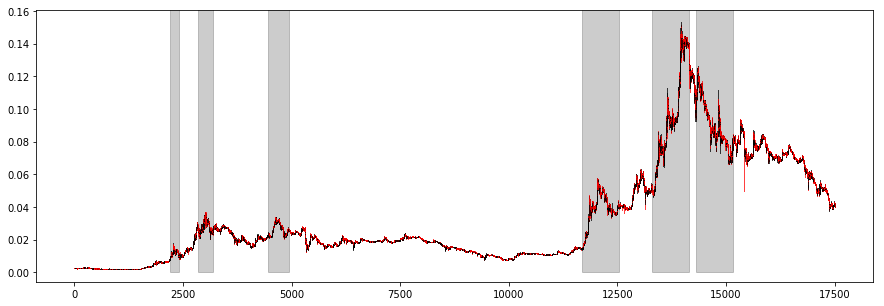Areas of increased volatility with potential correlation are highlighted above. Further assessment can be made by converting the series to percentage returns.

In :
_, ax = plt.subplots(figsize=(15,  5))
plt.plot(ethu['close'].pct_change().dropna())
area1 = plt.Rectangle((1450, -1), 600, 2, color='black', alpha=0.2)
area2 = plt.Rectangle((2150, -1), 1200, 2, color='black', alpha=0.2)
area3 = plt.Rectangle((3490, -1), 2100, 2, color='black', alpha=0.2)
area4 = plt.Rectangle((8700, -1), 2100, 2, color='black', alpha=0.2)
area5 = plt.Rectangle((11000, -1), 2100, 2, color='black', alpha=0.2)
plt.show()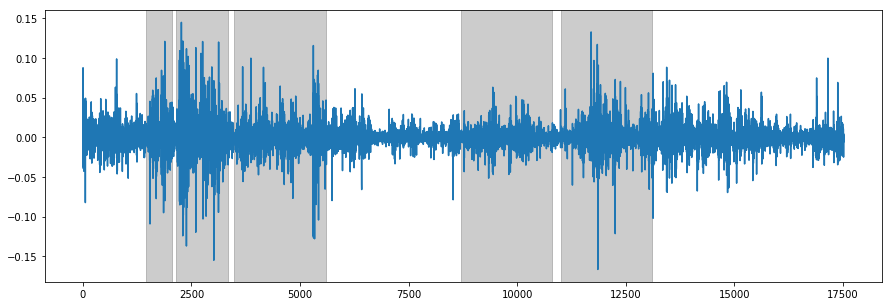The graph above shows some of the volatility clusters (this effect is known as heteroskedasticity) even more clearly. There's a strong implication of serial correlation. However, we should really perform an objective test for serial correlation. We can do this by choosing a holding window and a lookback window, iterating over the closing prices at every hour and computing the correlation coefficient and its p-value. An increasing correlation value and a small p-value (< 0.05 or the 95th percentile, allowing us to dismiss the null-hypothesis) suggests strong serial correlation in the series.

In :
import scipy.stats

def p_corr(df1, df2):
corr = df1.corr(df2)
N = np.sum(df1.notnull())
t = corr*np.sqrt((N-2)/(1-corr**2))
p = 1-scipy.stats.t.cdf(abs(t),N-2)  # one-tailed
return corr, t, p

pd.options.display.float_format = '{:,.11f}'.format

res = []
for lookback in [1, 5, 10, 25, 60, 120, 250]:
for holdhours in [1, 5, 10, 25, 60, 120, 250]:
df_Close_lookback = ethu.close.shift(lookback)
df_Close_holdhours = ethu.close.shift(-holdhours)
ethu['ret_lag'] = (ethu.close-df_Close_lookback)/df_Close_lookback
ethu['ret_fut'] = (df_Close_holdhours-ethu.close)/ethu.close
dfc = ethu[['ret_lag','ret_fut']].dropna()
idx = None
if lookback >= holdhours:
idx = np.array(range(0,len(dfc.ret_lag), holdhours))
else:
idx = np.array(range(0,len(dfc.ret_lag), lookback))
dfc = dfc.iloc[idx]
t, x, p = p_corr(dfc.ret_lag, dfc.ret_fut)
res.append([lookback, holdhours, t, p])
res = pd.DataFrame(res,columns=['lookback','holdhours','correlation','p value'])
print(res[res['lookback'] >= 10])

    lookback  holdhours    correlation       p value
14        10          1 -0.03335101396 0.00000507752
15        10          5 -0.02824556506 0.04736030273
16        10         10  0.02052663790 0.19539992633
17        10         25  0.07275953720 0.00116440493
18        10         60  0.06245129518 0.00453415305
19        10        120  0.06339368479 0.00409228486
20        10        250  0.04730735110 0.02470320336
21        25          1  0.00693489515 0.17952877813
22        25          5  0.03747651189 0.01332887191
23        25         10  0.06889845808 0.00197096501
24        25         25  0.05796220603 0.06288658997
25        25         60  0.06986453106 0.03253728122
26        25        120  0.05290023181 0.08179988978
27        25        250  0.06921368367 0.03461026230
28        60          1  0.00541163074 0.23730887569
29        60          5  0.03309830885 0.02526566576
30        60         10  0.06257574088 0.00446557658
31        60         25  0.07713320978 0.02081406056
32        60         60  0.08892304725 0.06542492473
33        60        120  0.02363002941 0.34456890619
34        60        250  0.07923982994 0.09034147592
35       120          1  0.00864397278 0.12712395278
36       120          5  0.03631159279 0.01610861249
37       120         10  0.06392591978 0.00383107173
38       120         25  0.04597990262 0.11302058082
39       120         60  0.02535500477 0.33387572910
40       120        120 -0.04316730261 0.30371949637
41       120        250  0.06543436958 0.21874274867
42       250          1  0.01132685125 0.06832585882
43       250          5  0.03560338417 0.01821746482
44       250         10  0.04934513341 0.02019125159
45       250         25  0.07039906381 0.03228867695
46       250         60  0.11083869974 0.03037476346
47       250        120  0.08192818536 0.16533717835
48       250        250  0.27025476220 0.01235642459


Notice the progressively increasing correlation and the fairly small p-values. We can test this further by designing a very simple strategy based on these results. We pick an acceptable lookback and p-value pair, enter a long if the cumulative returns over the lookback window are positive (this indicates momentum) and close the position as soon as the hold period expires.

In :
def calculateMaxDD(cumret):
highwatermark=np.zeros(len(cumret))
drawdown=np.zeros(len(cumret))
drawdownduration=np.zeros(len(cumret))
for t in range(1,len(cumret)):
highwatermark[t]=np.max([highwatermark[t-1], cumret[t]])
drawdown[t]=(1+cumret[t])/(1+highwatermark[t])-1
if (drawdown[t]==0):
drawdownduration[t]=0
else:
drawdownduration[t]=drawdownduration[t-1]+1
return np.min(drawdown), np.max(drawdownduration)

def report(df,lookback,holdhours):
longs = df.close > df.close.shift(lookback)
shorts = df.close < df.close.shift(lookback)
df['pos'] = 0.
for h in range(holdhours):
long_lag = longs.shift(h).fillna(False)
short_lag = shorts.shift(h).fillna(False)
df.loc[long_lag,'pos'] += 1
df.loc[short_lag,'pos'] -= 1

ret=(df.pos.shift(1)* (df.close-df.close.shift(1)) / df.close.shift(1)) / holdhours

cumret=np.cumprod(1+ret)-1

print('APR', ((np.prod(1.+ret))**(17500./len(ret)))-1)
print('Sharpe', np.sqrt(17500.)*np.mean(ret)/np.std(ret))
print('Drawdown', calculateMaxDD(np.array(cumret)))
return cumret

cumret=report(ethu, lookback = 60, holdhours = 5)

_, ax = plt.subplots(figsize=(15,  5))
plt.plot(cumret)
plt.show()

APR 49.6784523409
Sharpe 3.05714656552
Drawdown (-0.62736279599958034, 2973.0)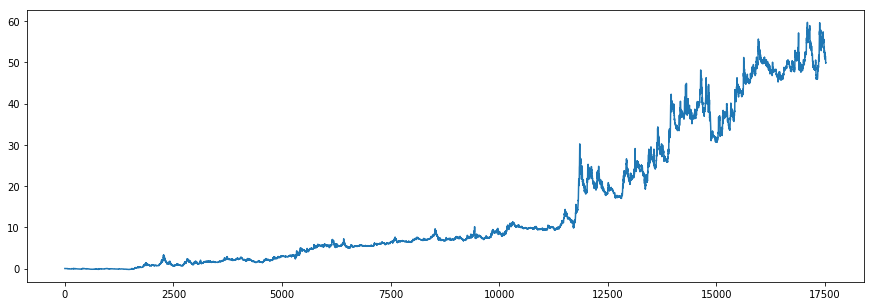Choosing a lookback window of 60 hours and a hold window of 5 hours (lowest p-value) nets an annual percentage rate of ~50%, not bad for such a terrible strategy! Of course the above is a rather simplistic approach to backtesting, for one we didn't take any fees into account and neither did we account for any slippage. A sharpe ratio of over 3 points is very optimistic. But nevertheless the above simply demonstrates the effects of correlation and momentum.

We can also visualize correlation directly by looking at correlogram plots. Below are two correlograms, the ETH/BTC price series to the left and a truly random normal series to the right. Notice the difference between the two plots.

In :
from statsmodels.graphics.tsaplots import plot_acf
_, axarr = plt.subplots(1, 2, figsize=(20, 10))
plot_acf(ethu['close'], axarr, title='ETH/BTC pair autocorrelation')
plot_acf(np.random.normal(1, 1, 1000), ax=axarr, title='Random Normal distribution autocorrelation')
plt.show()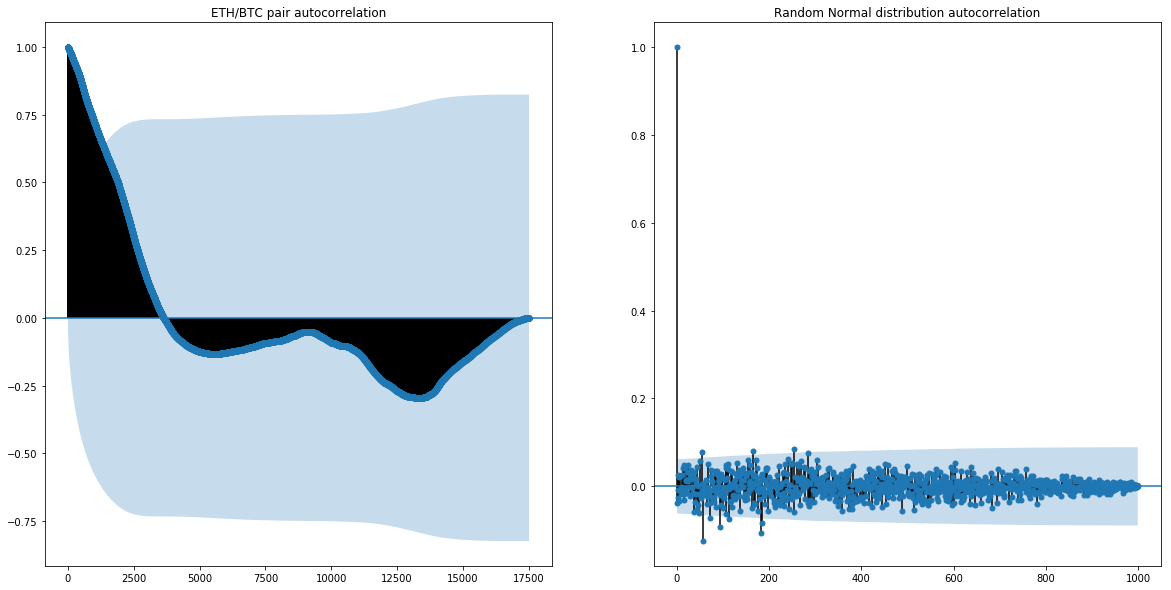## Signal and Noise¶

Once the presence of serial correlation has been established, we must next find a way to model it correctly. A perfect model is able to "explain away" the correlative random component of the time series. Lets imagine an artificial series which is dependent on time in such a way that the asset's price increases whenever the clock strikes midnight and decreases whenever the clock hits noon. An effective model is able to explain away the generative stochastic process behind the series (the clock in this example).

Another interpretation is that of signal and noise. We can look at a price distribution, for example the closing price series of an asset, as an observable, albeit noisy, signal. Our task is "smoothing" this signal, removing the noise so to say, to figure out the underlying process.

With the case of momentum we are really interested in the underlying trend. Rather than following every up and down, every bump and peak in a time series, we want to follow a clear trend, short- or longterm as early and as closely as possible.

#### Whats out there¶

Lets now look at some of the trend following algorithms available in the standard technical analysis toolbox. We will try to identify the underlying trend of the BCH/BTC pair. Below is a visualization of the hourly closing price series.

In :
df = pd.read_csv('/home/lisper/fintech/data/btc_bch_1h.csv')
[o, h, l, c] = [x.reshape(521, ) for x in np.split(df[['open', 'high', 'low', 'close']].values, 4, 1)]
_, ax = plt.subplots(figsize=(10, 5))
area1 = plt.Rectangle((70, 0), 25, 1, color='black', alpha=0.2)
area2 = plt.Rectangle((105, 0), 20, 1, color='black', alpha=0.2)
area3 = plt.Rectangle((153, 0), 10, 1, color='black', alpha=0.2)
area4 = plt.Rectangle((240, 0), 25, 1, color='black', alpha=0.2)
area5 = plt.Rectangle((372, 0), 36, 1, color='black', alpha=0.2)
plt.plot(c)
plt.show()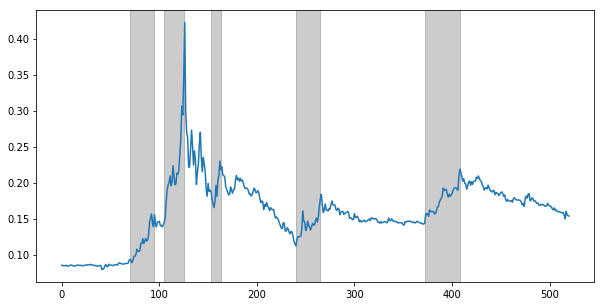Dismissing shorting opportunities for a moment, the highlighted areas above display some of the profitable longs via upward momentum trends.

#### Autoregression, Moving Average, Autoregressive Moving Average and the Autoregressive Integrated Moving Average¶

The majority of all trend following indicators in use right now are based on four simple models:

1. The Autoregressive Model of Order p (AR)
2. The Moving Average of Order q (MA)
3. The Autoregressive Moving Average Model of Order pq (ARMA)
4. The Autoregressive Integrated Moving Average of Order pdq (ARIMA)

$$x_t = \sum_{i=1}^p{\alpha_ix_{t-1} + w_i} \tag{Autoregressive Model of order p} \text{ } \text{where w_t is white noise and \alpha_i \in \mathbb{R}}$$

Essentially the autoregressive model is simply an extension of the random walk (the random walk is defined as: $x_t = x_{t-1} + w_t$). It is a linear model where each new term $x_t$ depends linearly on previous terms $x_{t-1}$ with coefficients for each term $\alpha_i$ and a random noise component. The coefficients must be selected manually. Intuitively, the autoregressive model captures "shock" or "noise" through a regression process; each new term is the addition of noisy previous terms.

$$x_t = w_t + \sum_{i=1}^q{\beta_i w_{t-q}} \tag{Moving Average Model of order q} \text{ } \text{where w_t is white noise and \beta_i \in \mathbb{R}}$$

The moving average model is very similar to the autoregressive model, only instead of being a combination of past time series values it is a combination of past "white noise" or "shock" values. In other words, it captures noise on the direct rather than indirect level.

Now the ARMA and ARIMA models are simply a combination of the previous two models with some additions. The ARMA model is able to capture noise directly (as an additive process like the MA model does) and indirectly through the addition of previous terms (like the AR model does). The ARIMA model is ARMA (direct and indirect capture of noise) with the addition of an integrated component; meaning the time series is integrated $d$ times by the model in order to de-noise it of seasonal trends, heteroskedastic clusters, etc before the regular ARMA model is applied.

#### Evaluating Performance¶

Below is a visualization of various AR, MA, ARMA and ARIMA based indicators applied to our BCH/BTC series.

In :
import talib

_, ax = plt.subplots(3, 2, figsize=(30, 25))
inds = [
('EMA', talib.EMA(c, 7)),
('DEMA', talib.DEMA(c, 7)),
('HT', talib.HT_TRENDLINE(c)),
('KAMA', talib.KAMA(c, timeperiod=7)),
('T3', talib.T3(c, timeperiod=7, vfactor=1)),
('VMA', talib.WMA(c, timeperiod=7))
]

for j in range(0, 3):
for i in range(0, 2):
name, ind = inds.pop()
ax[j][i].plot(ind, label=name, color='r')
ax[j][i].plot(c, label='Actual', linestyle=':')
ax[j][i].legend()
plt.show()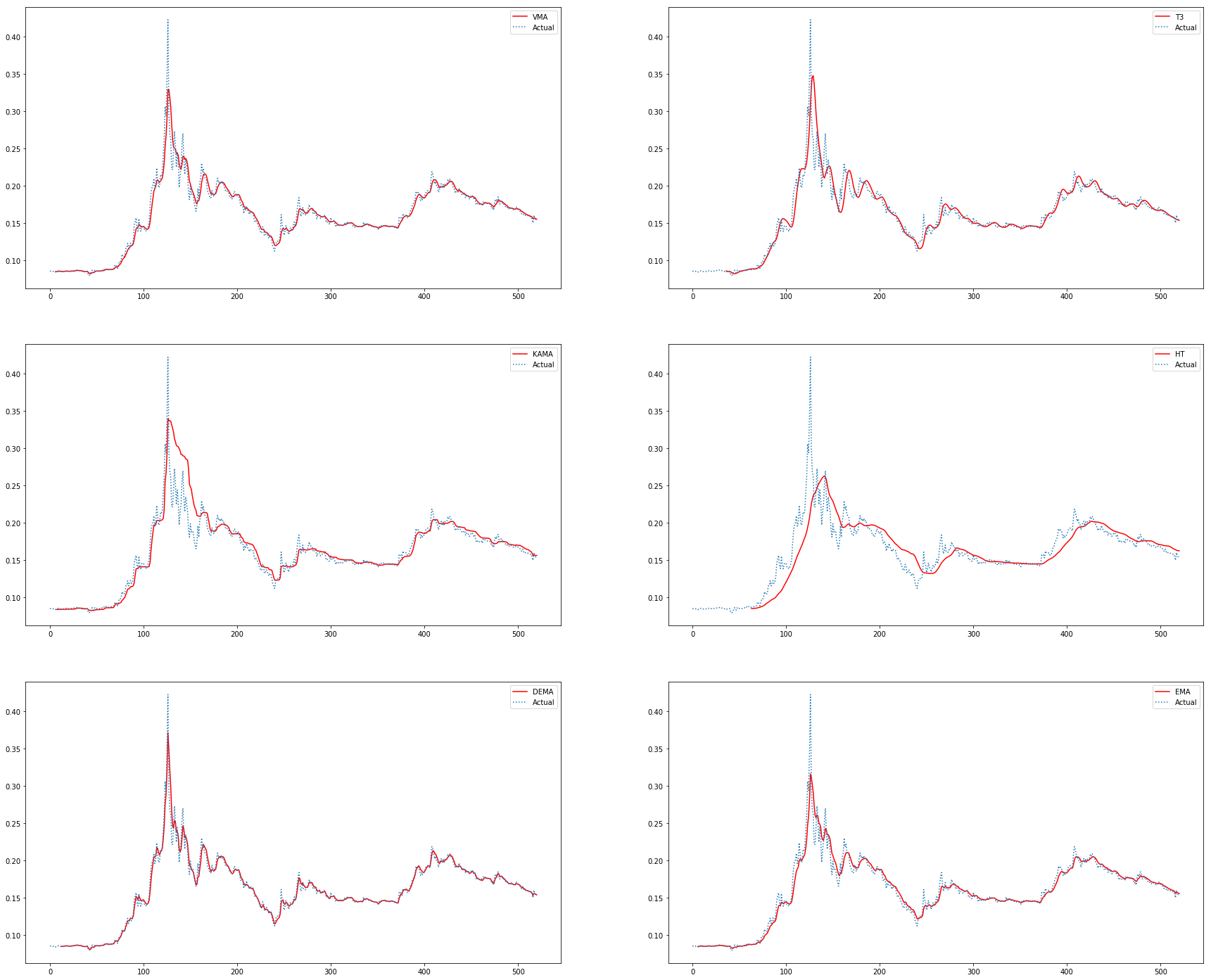Upon first inspection all indicators capture an underlying trend to various degrees of success. However, upon closer inspection a number of issues should become apparent. For one, as in the case of the T3, EMA, HT and KAMA indicators, lag is a persistent problem. The model does not react to the underlying change of trend in due time. DEMA on the other hand captures the trend in time, however the resulting "smooth" signal is itself quite noisy plagued by various bumps and peaks. Over- and undershooting or over- and underreaction are also a problem area.

Evaluating the results above we can simply conclude that our models are simply unable to correctly capture noise and explain it away via regression, moving averages and so on.

An issue with all the models described above is their treatment of noise at large. Noise is viewed in a somewhat static manner, it is clear that we must represent noise itself through some stochastic process and find a model able to adjust the representation of noise as it is processing it.

## The State-Space Model¶

The general premise of a state space model is that we have a set of states that evolve in time (for example momentum at time $t_{i-1}$ somehow changes and evolves to momentum at time $t_i$), but our observations of these states contain statistical noise (such as market infrastructure noise, irrational behaviour by participants, etc.) and hence we are unable to ever observe the "true" state. The goal of the state space model is to infer information about the states (for example identifying a trend), given the observations, as new information arrives.

State space models are also very general and is possible to put all of the models evaluated above into a state space formulation thus making them more dynamic.

The state space model is given by the following relationship

$$\theta_t = G_t \theta_{t-1} + w_t \tag{State equation}$$ $$Y_t = F_t^T \theta_t + v_t \tag{Observation equation} \text{, where F^T is the matrix transpose of F}$$

The state space model represents hidden states at time $t$: $\theta_t$ as a linear combination of previous states $\theta_{t-1}$ transformed by a time varying matrix $G_t$ as well as system noise $w_t$. Both of the transformation elements $G_t$, $w_t$, and how those elements change over time, can be specified by us. Similarly, the observations $y_t$ (that is, something we can directly measure, like the price level) are a linear combination of current, hidden state $\theta_t$ and an additional random variation, the measurement noise $v_t$.

Intuitively one can view the state space model as an approximator of hidden state under uncertainty. For example we can imagine a faulty tracking device that provides us with noisy, error filled measurements of a rocket's position and we are interested in its velocity at any given time. As we receive more and more inputs from our error prone measuring device we can estimate the true state, the actual velocity of the rocket, with greater and greater certainty, gradually explaining away all the noise in our system.

#### The Kalman Filter¶

The Kalman filter is a state-space algorithm derived from the general state-space model relationship. It is most commonly found in aeronautics, guidance and navigation of various rockets, spacecraft trajectory analysis and various other engineering tasks. However, since we have established the price of an asset as a measurable signal of its underlying trend and momentum we can use the Kalman filter to help us model this relationship.

The kalman filter is defined through a series of equations

$$X_{t+1} = \Phi X_t + c_t + w_t \tag{State equation}$$ $$Y_t = HX_t + d_t + v_t \tag{Observation equation}$$ $$X_{t+1|t} = \Phi X_{t|t} + c_t \tag{Prediction}$$ $$X_{t+1} = X_{t+1|t} + K_{t+1}(Y_{t+1} - Y_{t+1|t}) \tag{Correction}$$ $$K_{t+1} = P_{t+1|t} H^T[HP_{t+1|t}H^T + R]^{-1} \tag{Kalman gain}$$

Hidden state is once again represented as a linear combination of previous states, transformed by matrix $\Phi$, system noise $w_t$ as well as an optional correction vector $c_t$. Similarly, observations are represented as the transform of current state via transformation matrix $H$, measurement noise $v_t$ as well as the addition of a measurement correction vector $d_t$. Once the filter is specified new states are drawn via the prediction equation, once a new measurement is available subsequent predictions can be corrected via the correction and kalman gain relationship.

As before the kalman filter can be used to specify all previous models in state-space form. It should also be noted that while the kalman filter is a linear-dynamic system it is still able to model and measure non-linear behaviour. This property makes the kalman filter especially attractive, as relying on non-linear models alone (such as neural-networks and other machine learning objects) introduces many complexities into the system, while a purely linear model can be easily debugged and dealt with.

#### Model specification under the Kalman Filter¶

Now it is worth remembering that the kalman filter itself is just an algorithm which allows us to make better estimations of hidden states when dealing with noisy, uncertain measurements. The model specification, in other words parameters $\Phi$, $v$, $H$, $w$ and so on are all up to us to define and specify. While this does give us a great degree of freedom, nothing comes for free and estimating initial parameters can be rather difficult. For example, the kalman equation asks us to provide an initial estimate of system noise at $t_0$: $w_0$, how this estimate is derived and defined is entirely up to us.

Practically speaking, to make effective use of the filter we must have an idea of the underlying behaviour of our system. At least, we should have an approximate understanding of what it is we are trying to model. To draw upon an example, if we were modelling the beheaviour of a B52 bomber and the effects of turbulent weather on its velocity we would probability specify the whole model, noise, etc using classical mechanics.

When it comes to financial modelling things are not as clear cut.

That being said, we can make use of other algorithms especially those popular in genetic programming like "Particle Swarm Optimization" to tune our filter and derive the whole model from available data itself.

## Modelling in State-Space¶

I hope to have provided sufficient theoretical background to build intuition of the modelling task at hand. Next, we will consider, develop and backtest several dyanmic linear systems under the state-space framework. The rest of this notebook will discuss the application of these models, directly to spot trend and momentum as well as indirectly as a preprocessing step for various other models.

#### Price series and word of caution¶

Since we are interested in exploiting momentum, we should test our models on a somewhat volatile but not too volatile series. Ideally we are interested in various price regimes: upswings, downswings as well as flat movement. For example, there would be little point in testing against a constantly uptrending pair like BTC/USDT since even a completely terrible model would probably end up locking in some profits. Similarly, we do want momentum, so theres no point in testing against a 95% downtrending market. For this reason, I choose the familiar BCH/BTC pairing from above. Below is the 5min OHLC chart, starting early AUG and ending late NOV.

In :
import pandas as pd
import matplotlib.pyplot as plt
from mpl_finance import candlestick2_ochl

_, ax = plt.subplots(figsize=(20,  5))
candlestick2_ochl(ax, s['open'], s['close'], s['high'], s['low'], width=2)
plt.show()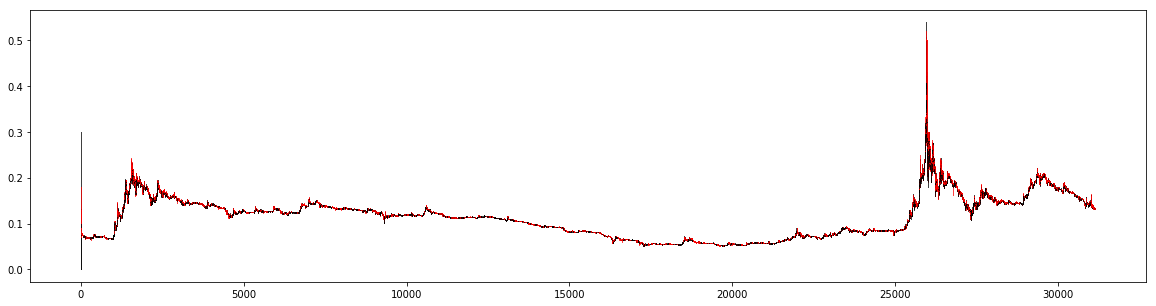Theres some huge volatility spikes at the start as well as towards the end of the series. A prolonged period of sideways movement is also present. This should give us plenty of data-points for a fairly well rounded test against several market conditions. One final word of caution applies. We should be aware about introducing inherent bias into all of our tests: the presence of momentum is already known to us! In a live scenario for example the presence of those volatility spikes found towards the end of the series would be entirely unknown to us, the sideways movement could continue for years to come. Now relatively speaking, this is a minor point as far as tests themselves are concerned. As long as we treat tests in a completely transparent fashion, making sure the algorithm has no way to "look into the future", etc we will effectively simulate a real scenario. However, we should be aware that momentum is not a given and market conditions can change drastically. In a real setting, to guard against running a momentum model in a non-momentum market, we would supplement our model with a regime-switch model, but more on that later.

#### Benchmark¶

Next, lets define a model benchmark. We are not really interested in profit and loss as such, because it is a rather simplistic view of performance. As I mentioned previously, in boom market conditions even the most terrible model will be profitable. Rather, we are interested in outperforming the base market. While traditionally we could just compare our model against an index benchmark (the S&P 500 for example) no such thing is currently available for crypto markets.

We could go ahead and construct our own bucket of base crypto currencies and test against that but this is probably overkill for our simple scenario. Instead, we will test against a "buy and hold" strategy. Here we buy the asset at time $t_0$ and never sell it. Starting balance is \$100. Backtest is shown below.

In [ ]:
! backtrader ../backtests/buy_and_hold.py ../backtest_graphs/buy_and_hold.png

In :
from IPython.display import Image, display
from IPython.core.display import HTML